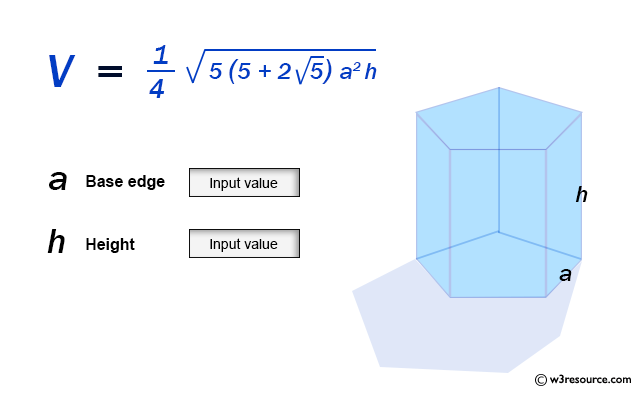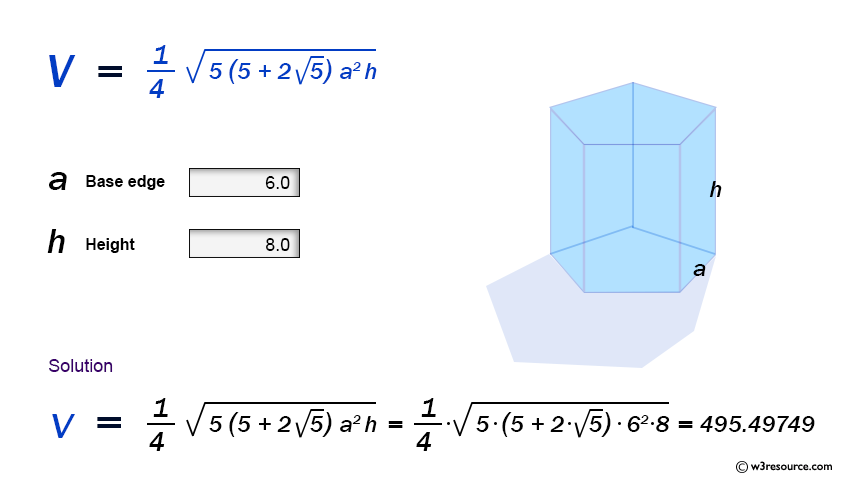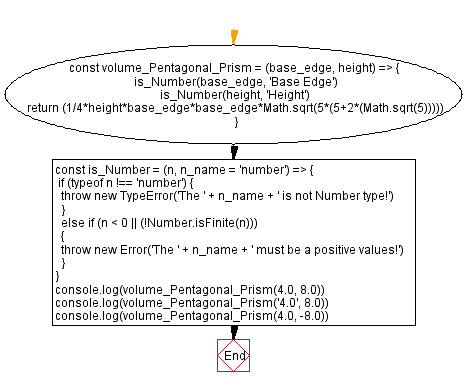# JavaScript: Volume of a Pentagonal Prism

## JavaScript Math: Exercise-62 with Solution

Write a JavaScript program to calculate the volume of a prism using only its height and one of its hexagonal sides.

In geometry, the pentagonal prism is a prism with a pentagonal base. It is a type of heptahedron with seven faces, fifteen edges, and ten vertices.

Sample Data:Sample Solution:

HTML Code:

``````<!DOCTYPE html>
<html>
<meta charset="utf-8">
<title>JavaScript function to Volume of a Pentagonal Prism</title>
<body>

</body>
</html>
```
```

JavaScript Code:

``````const volume_Pentagonal_Prism = (base_edge, height) => {
is_Number(base_edge, 'Base Edge')
is_Number(height, 'Height')
return (1/4*height*base_edge*base_edge*Math.sqrt(5*(5+2*(Math.sqrt(5)))))
}

const is_Number = (n, n_name = 'number') => {
if (typeof n !== 'number') {
throw new TypeError('The ' + n_name + ' is not Number type!')
}
else if (n < 0 || (!Number.isFinite(n)))
{
throw new Error('The ' + n_name + ' must be a positive values!')
}
}
console.log(volume_Pentagonal_Prism(4.0, 8.0))
console.log(volume_Pentagonal_Prism('4.0', 8.0))
console.log(volume_Pentagonal_Prism(4.0, -8.0))
```
```

Sample Output:

```220.22110727538777
-----------------------------------------------------------
Uncaught TypeError: The Base Edge is not Number type!
at https://cdpn.io/cpe/boomboom/pen.js?key=pen.js-5d61fb21-ef72-d840-7127-0d851f83a866:9
-----------------------------------------------------------------------------------------
Uncaught Error: The Height must be a positive values!
at https://cdpn.io/cpe/boomboom/pen.js?key=pen.js-efb93b94-2200-1f40-7134-9a617d9067bc:13

```

Flowchart:Live Demo:

See the Pen javascript-math-exercise-62 by w3resource (@w3resource) on CodePen.

Improve this sample solution and post your code through Disqus

Previous: Volume of a Triangular Prism.
Next: Volume of a Sphere

What is the difficulty level of this exercise?

Test your Programming skills with w3resource's quiz.

﻿